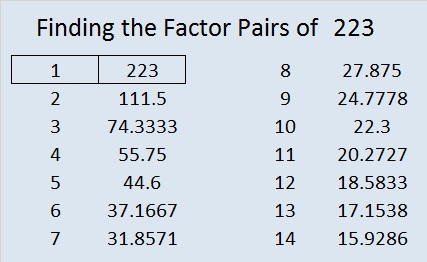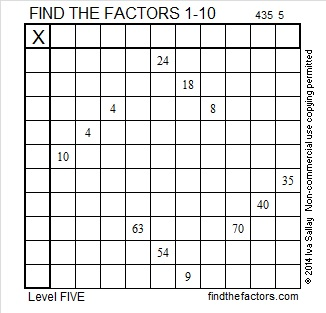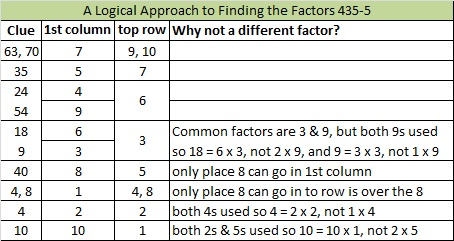# 223 and Level 5

• 223 is a prime number.
• Prime factorization: 223 is prime.
• The exponent of prime number 223 is 1. Adding 1 to that exponent we get (1 + 1) = 2. Therefore 223 has exactly 2 factors.
• Factors of 223: 1, 223
• Factor pairs: 223 = 1 x 223
• 223 has no square factors so its square root cannot be simplified. √223 ≈ 14.933How do we know that 223 is a prime number? If 223 were not a prime number, then it would be divisible by at least one prime number less than or equal to √223 ≈ 14.933. Since 223 cannot be divided evenly by 2, 3, 5, 7, 11, or 13, we know that 223 is a prime number.Excel file of puzzles and previous week’s factor solutions: 10 Factors 2014-09-01